## Saturday, January 30, 2010

### Diffraction of waves

When a waves meets a barrier or passes through a gap, the wave will bend around the edges or diffracted. The diffractrion effect is more obvoius when the size of the gap or barrier is about the same size of the wavelength.

When waves are diffracted, the direction of propagation and shape of the waves change while the wavelength, frequency and speed remains the same.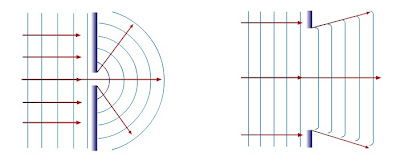If the gap is bigger than the wavelength,
i ) the waves in the centre go through the gap unchanged
ii) the waves at the edges are diffracted.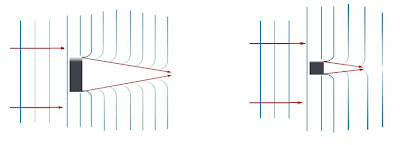If the barrier is bigger that the wavelength, a large shadow area is formed behind the barrier. the diffraction pattern of the waves is less obvious.

The pattern of light diffraction is not easily seen because it wavelength is small ( x 10 -7 m.

## Friday, January 29, 2010

### Selamat Menyambut Thaipusam ( Happy Thaipusam )I wish a Happy Thaipusam to all who celebrate it...

## Thursday, January 28, 2010

### The Thin Lens Equation

We can ﬁnd the position of the image of a lens mathematically as there is mathematical relation between the object distance, image distance, and focal length.where
u = object distance [cm]
v = image distance [cm]
f = focal length of lens [cm]

When applying the equation, take note of the following:
1) The object distance,u, is always positive.

2) If the image on the same side of the lens as the light leaves the lens. Then v is positive and the image is real .

3. If the image on the same side of the lens as the light rays enter the lens. Then v is negative
and the image is virtual.

Worked example :

1. An object of height 3 cm is placed
(a) at 30 cm
(b) at 5 cm
from a convex lens of focal length 10 cm. determine the position and the size of the image in each case.

solution :
given ,
u= 30 cm, f = 10 cm

find v=?

1/f = 1/u + 1/v
1/10 = 1/30 + 1/v
v= +15 cm
(a positive sign shows that the image is real )

m = image height / object height = v/u
image height / 3 = 15/30
==> image height =1.5 cm

The image is real and at the distance of 15 cm from the lens on the opposite side of the object. the height of the image is 1.5 cm.

## Wednesday, January 27, 2010

### Drawing Ray Diagrams for Converging Lenses

There's an interactive simulation available on the "Learning by Simulations" Web site which allows to experiment with various lenses and different focus lengths. http://www.vias.org/simulations/simusoft_lenses.html

Convex lens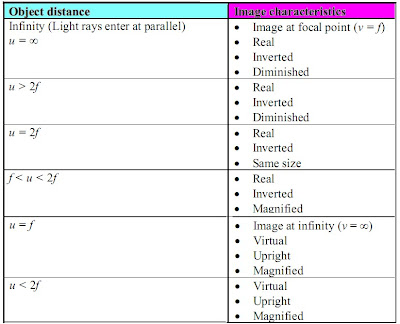Concave lensImage characteristics:
• Virtual
• Upright
• Diminished

## Tuesday, January 26, 2010

### The power of lensesThe power of lens is a measure of its ability to converge or diverge an incident beam of light.
It is defined as the reciprocal of the focal length, in meters .
The unit of power is m-1 or dioptre.

Optical power
(also referred to as dioptric power, refractive power, focusing power, or convergence power)
Sourse : http://en.wikipedia.org/wiki/Lens_power

Worked example :
Determine the power of each of the following lenses.
a) Convex lens, f = 10 cm,
b) Concave lens, f = 10 cm.

Solution :
a) power,P = 1/0.1
= 10 D

b) power , P = -1/0.1
= -10 D

## Monday, January 25, 2010

### Spherical lens and cylindrical lens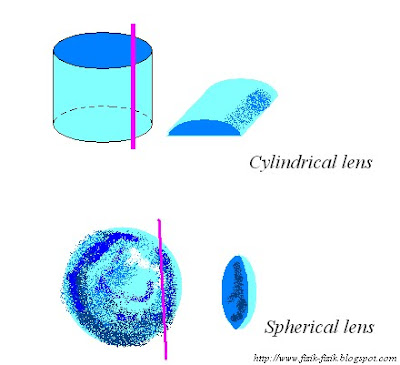--> A cylindrical lens plano-convex lens is made by cutting trough a side of a cylinder.
--> A spherical plano-convex lens is made by cutting through a portion of a sphere.

### Ghost ship (Superior mirage)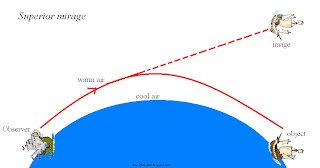A mirage can be also observed at the sea in the vicinity of the geographical pole.

In this area, the upper layers of air are hot compared to the lower layers of the air.
Rays of the ship are refracted away from the normal until total internal refraction occurs. After that, the rays of light are refracted following a curve bending towards the normal until they reach the eyes of the observer.
The image is inverted and appears in the sky.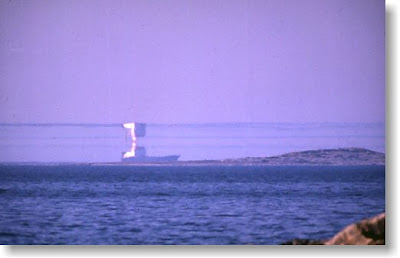Superior mirage of tanker in Finland (http://virtual.finland.fi/finfo/english/mirage.html).The Superior Mirage of Iceburg (http://www.phys.ufl.edu/~avery/course/3400/gallery)

## Saturday, January 23, 2010

### Properties of lenses

Thin lenses are divided into:

a) Converging lenses

b) Diverging lensesBefore we study lenses in detail, there are a few important terms that must be deﬁned.

The principal axis is the line which runs horizontally straight through the optical centre of the lens. It is also sometimes called the optic axis.

The optical centre (O) of a convex lens is usually the centre point of the lens. The direction of all light rays which pass through the optical centre, remains unchanged.

The focus or focal point of the lens is the position on the principal axis where all light rays which run parallel to the principal axis through the lens converge (come together) at a point.
(Note: the plural form of the word focus is foci.)

• The focal length (f) is the distance between the optical centre and the focal point.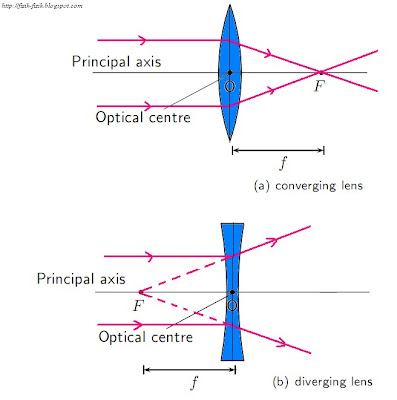## Friday, January 22, 2010

### Lens

A lens is any transparent material (e.g. glass) of an appropriate shape that can take parallel rays of incident light and either converge the rays to a point or diverge the rays from a point.

Lenses change the direction of light rays by refraction.
Lenses are used in eyeglasses, cameras, microscopes, and telescopes. You also have lenses in your eyes!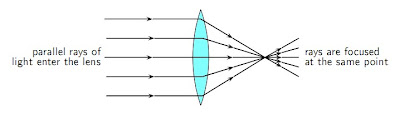Converging Lenses (positive lenses)
Converging lenses converge parallel rays of light and are thicker in the middle than at the edges.
Converging or concave lens focus light rays to a single point.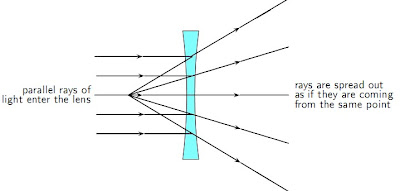Diverging Lenses (negative lenses)
Diverging lenses diverge parallel rays of light and are thicker at the edges than in the middle.
Diverging or convex lenses spread out the light rays so that it looks like they all come from the same point.

## Thursday, January 21, 2010

### AquariumDue to the total internal refraction, part of the water surface acts as a perfect mirror, which allows the fish and the diver to see objects in the water and the object around the obstacles.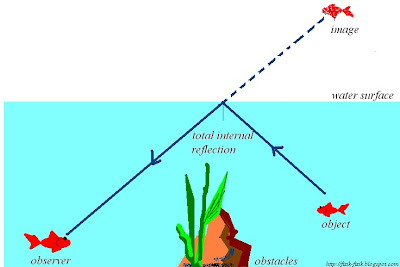Find our more :

Formation of rainbow== http://www.rebeccapaton.net/rainbows/formatn.htm#diagram

## Wednesday, January 20, 2010

### The Electromagnetic Spectrum Song - by Emerson & Wong Yann (Singapore)

Hari ini kami menyanyi, lupakan latihan, nota dan kiri-kira anda.
Pesanan Pn.Chow:" Jangan nyanyi lagu ini semasa uji atau peperiksaan !!"(nyanyi dalam hati saja okey ?)

### Water ! Water !! ( Inferior Mirage )

Mirage = An optical phenomenon that creates the illusion of water, often with inverted reflections of distant objects, and results from distortion of light by alternate layers of hot and cool air. Also called fata morgana.While driving a car on a hot day, you may see a pool of water on the road. But as you approach that particular spot, you find that the pool does not really exits.

The above occurrence is called mirage. It is caused by refraction and total internal reflection.

Mirages normally occur in the daytime when the weather is hot.
The hot air near the ground surface warms the air above it causing a light ray passing through it to be refracted upwards. Because the light ray reaching the viewer's eye appears to be coming from the ground surface, and because the reflected ray follows the same path, an apparent image is seen.

Other inferior Mirage phenomena:
1. Sunset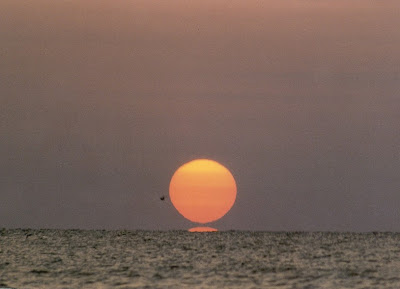photo source : http://mintaka.sdsu.edu/GF/explain/simulations/inf-mir/Kaplan_photos.html
The miraged image of the Sun's (lower edge).

## Monday, January 18, 2010

### Optical fiber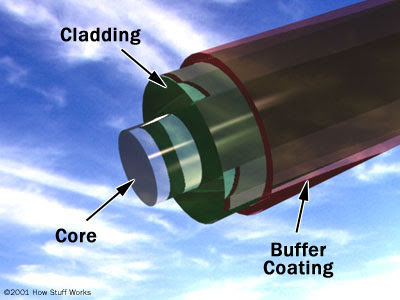Photo source : How Stuff Work

An optical fiber (or fibre) is a glass or plastic fiber that carries light along its length.

Light is kept in the core of the optical fiber by total internal reflection.

Fiber optics has several advantages over traditional metal communication lines:

• Fiber optic cables have a much greater bandwidth than metal cables. This means that they can carry more data.
• Fiber optic cables are less susceptible than metal cables to interference.
• Fiber optic cables are much thinner and lighter than metal wires.
• Data can be transmitted digitally rather than analogically.

The main disadvantage of fiber optics is that the cables are expensive to install. In addition, they are more fragile than wire and are difficult to splice.

## Saturday, January 16, 2010

### From refraction to total internal reflection

1. The angle of the incident light= 0◦.
The angle of refraction = 0◦2. The angle of the incident light more than 0◦ and less than critical angle θc.
The angle of refraction less than 90◦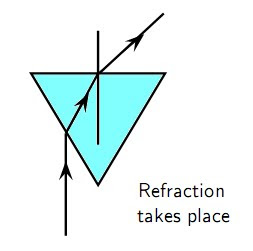3. The angle of the incident light is called the critical angle, θc, when the angle of reﬂection is 90◦4. The angle of incident light larger than the critical angle, the refracted ray runs along the surface of the medium. This is called total internal reﬂection .### Form 3 Science Chapter 4 (Reproduction)

Dear form 3 students, you just need to press "download" to download the document .
Form 3 Science Chapter 4

## Friday, January 15, 2010

### Total Internal Reﬂection

Total internal reﬂection takes place when light is reﬂected back into the medium because the angle of incidence is greater than the critical angle.

Each medium has its own unique critical angle. For example, the critical angle for glass is 42◦,
and that of water is 48.8◦. We can calculate the critical angle for any medium.Calculating the Critical Angle

Now we shall learn how to derive the value of the critical angle for two given media. The process is fairly simple and involves just the use of Snell’s Law that we have already studied.

To recap, Snell’s Law states:
n1 sin θ1 = n2 sin θ2

where n1 is the refractive index of material 1, n2 is the refractive index of material 2, θ1 is the angle of incidence and θ2 is the angle of refraction.

For total internal reﬂection we know that the angle of incidence is the critical angle.
So,
θ1 = θc.

However, we also know that the angle of refraction at the critical angle is 90◦. So we have:

θ2 = 90◦

We can then write Snell’s Law as:

n1 sin θ1 = n2 sin 90◦

Important:
Take care that for total internal reﬂection the incident ray is always in the denser medium.Virtual Lab :

http://www.learnerstv.com/animation/animation.php?ani=102&cat=physics

## Thursday, January 14, 2010

### Happy Ponggal"Wishing that this festival brings good luck and prosperity and hoping that it is joyous, and fills your days ahead with happiness.
Have a wonderful Pongal."

### At The Beach

Berikut merupakan jawapan untuk muka surat 14. ( Tingkatan 5 )The figure shows water waves from the sea advancing towards a bay and a cape.

a) Why are the speed and wavelength of waves in the middle of the sea almost uniform?

b) Why do the distances between the wavefronts decrease as the waves approach the beach?

c) Why is the water in the bay stationary compared at the cape ?

a) The depth of the water in the middle of the sea is almost uniform.

b) On approaching the beach, the depth of the water decreases. The speed of the waves decrease and the wavelength is decreased.

c) The depth of the of the water varies across the area of he bay and the energy of the water wave spreads to wider area as compared to the he region near the cape.
( refer to the figure about )
The amplitude of the water waves near the bay is low and hence the water at the bay is comparatively still.

## Wednesday, January 13, 2010

### Critical Angle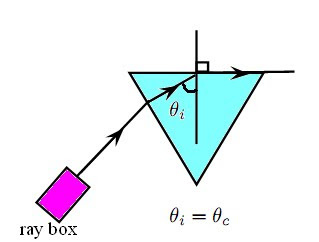The critical angle is the angle of incidence where the angle of reﬂection is 90◦.
The light must shine from a dense to a less dense medium.

If the angle of incidence is bigger than this critical angle, the refracted ray will not emerge from the medium, but will be reﬂected back into the medium. This is called total internal reﬂection.

Total internal reﬂection takes place when
• light shines from an optically denser medium to an optically less dense medium.
• the angle of incidence is greater than the critical angle.

## Monday, January 11, 2010

### Getting bigger or smaller ?

Khas untuk murid 5um and 5 ukm..

In any optical system where images are formed from objects, the ratio of the image height, hi, to the object height, ho is known as the magniﬁcation, m.

m = hi/ho

For a plane mirror, the height of the image is the same as the height of the object, so the magniﬁcation is simply m = hi/ho = 1.

If the magniﬁcation is greater than 1,
the image is larger than the object and is said to be magniﬁed.

If the magniﬁcation is less than 1, the image is smaller than the object so the image is said to be diminished.

Example:
Question:
A concave mirror forms an image that is 3.6 cm high. The height of the object is 1.2 cm. Calculate the magniﬁcation of the mirror.

Suggested solution,

Step 1 : Identify what is given and what is asked.
Image height hi = 3.6 cm
Object height ho = 1.2 cm
Magniﬁcation m = ?

Step 2 : Substitute the values and calculate m.
m = hi/ho
= 4.8/1.6
= 3
The magniﬁcation is 3 times.

## Saturday, January 9, 2010

### Illusion ?

Kepada murid 5Um dan 5Ukm yang mengambil matapelajaran fizik, sila ikuti siri "light" . Maaf saya tiada masa untuk membuat ulangkaji bahagian tingkatan 4 dengan anda setakat ini, kami perlu menghabiskan syllabus tingkatan 5 sebelum percubaan pertama yang dijangka diadakan pada bulan ogos.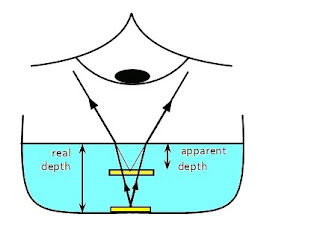Imagine a coin on the bottom of a shallow pool of water. If you reach for the coin, you will miss it because the light rays from the coin are refracted at the water’s surface.

Consider a light ray that travels from an underwater object to your eye. The ray is refracted at the water surface and then reaches your eye. Your eye does not know Snell’s Law; it assumes light rays travel in straight lines. Your eye therefore sees the image of the at coin shallower location. This shallower location is known as the apparent depth.

The refractive index of a medium can also be expressed as
n = real depth/apparent depth
n = refractive index ( without unit)

Let pratice:

1. A coin is placed at the bottom of a 40 cm deep pond. The refractive index for water is 1.33. How deep does the coin appear to be?

2. A coin appears to be 7 cm deep in a colourless liquid. The depth of the liquid is 10.43 cm.
a. Determine the refractive index of the liquid.
b. Identify the liquid.

3. A coin is placed in a bowl of acetone (n = 1.36). The coin appears to be 10 cm deep. What is the depth of the acetone?

4. A dot is drawn on a piece of paper and a glass prism placed on the dot according to the diagram.(a) Use the information supplied to determine the refractive index of glass.
(b) Draw a ray diagram to explain how the image of the dot is above where the dot really is.

### Which is easier to travel through, air or water?

People usually travel faster through air. So does light!

Deﬁnition: Refractive Index
The refractive index (symbol n) of a material is the ratio of the speed of light in a vacuum to its speed in the material and gives an indication of how diﬃcult it is for light to get through the material.

n = c / v
where
n = refractive index (no unit)
c = speed of light in a vacuum (3 × 108m s−1)
v = speed of light in a given medium (m s−1)

If the refractive index n increases, the speed of light in the material v must decrease. Light therefore travels slowly through materials of high n.

Light travels slower in any material than it does in a vacuum, so all values for n are greater than 1.Refractive indices of some materials.

Quiz:
1. Describe what is meant by the refractive index of a medium.

2. When light travels from air to a medium, its speed is decreased by 25%. What is the refractive index of the medium ?
(ans : Q2 = 1.33)

3. Light travels from a medium with n = 1,63 into a medium of n = 1,42.
(a) What happens to the speed of the light? Does it increase, decrease, or remain the same?
(b) Does the light bend towards the normal, away from the normal, or not at all?

## Wednesday, January 6, 2010

### Snell’s Law

The angles of incidence and refraction when light travels from one medium to another can be calculated using Snell’s Law.

Deﬁnition: Snell’s Law
n1sin θ1 = n2 sin θ2
where
n1 = Refractive index of material 1
n2 = Refractive index of material 2
θ1 = Angle of incidence
θ2 = Angle of refraction

Remember that angles of incidence and refraction are measured from the normal, which is an imaginary line perpendicular to the surface.If
n2 <>1
then from Snell’s Law,
sin θ1 < style="font-style: italic;">θ2.
For angles smaller than 90◦, sin θ increases as θ increases. Therefore,
θ1 < θ2.
This means that the angle of incidence is less than the angle of refraction and the light ray is away toward the normal.Similarly,if
n2 > n1
then from Snell’s Law,
sin θ1 > sin θ2.
For angles smaller than 90◦, sin θ increases as θ increases. Therefore,
θ1 > θ2.
This means that the angle of incidence is greater than the angle of refraction and the light ray is bent toward the normal.

What happens to a ray that lies along the normal line?

Worked Example : Using Snell’s Law

Question:
A light ray with an angle of incidence of 35◦ passes from water to air.
Find the angle of refraction using Snell’s Law . Discuss the meaning of your answer.
(the refractive index is 1,333 for water and about 1 for air)

According to Snell’s Law: n1sin θ1 = n2 sin θ2 1.33 sin 35◦ = 1 sin θ2 sin θ2 = 0.763 θ2 = 49.7◦ The light ray passes from a medium of high refractive index to one of low refractive index. Therefore, the light ray is bent away from the normal.

Test your understanding :
1. A light ray passes from water to diamond with an angle of incidence of 75◦. Calculate the angle of refraction. Discuss the meaning of your answer.
(Answer: 32.1◦, ....bent towards the normal)

## Monday, January 4, 2010

### What happens when light passes through a medium?

Refraction is the bending of light that occurs because light travels at diﬀerent speeds in diﬀerent materials.
When light moves from one medium into another (for example, from air to glass), the speed of light changes. The eﬀect is that the light ray passing into a new medium is refracted, or bent.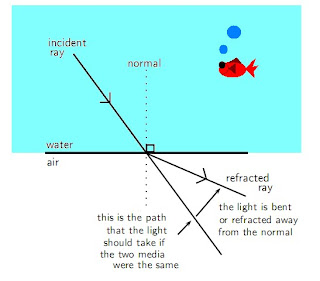When it travels from an optically dense medium like water or glass to a less dense medium like air, it will be refracted away from the normal .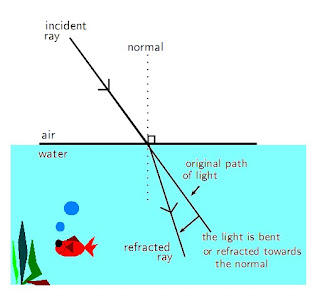If it travels from a less dense medium to a denser one, it will be refracted towards the normal.

Find out more:
1. what happened to light the light ray if it luminous perpendicularly to the surface of two different medium ?

## Sunday, January 3, 2010

### Buka Sekolah

Sudah lama bercuti, masih tak ada mood balik ke sekolah ?
Jagan cuba ponteng, belajarlah bersungguh-sungguh untuk masa depan yang cerah.
Selamat balik kesekolah...

Kira- kira :
1. Berapa jamkah anda berada di sekolah setiap hari ?
2. Purata masa tidur anda setiap hari ?
3. Bandingkan masa tidur anda dengan waktu sekolah anda, terkejutkan ?
4. Tolong jaga kebersihan sekolah (tentulah termasuk kelas dan tandas sekolah).Anda mauhukan katil anda selesa dan bersih, begitu juga keadaan sekolah.

Selamat maju jaya.